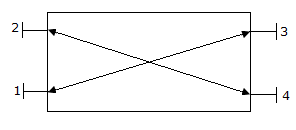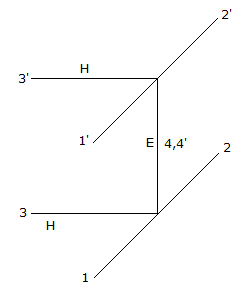# Electronics and Communication Engineering - Microwave Communication

### Exercise :: Microwave Communication - Section 8

46.

A waveguide twist is used to change the plane of polarization.

 A. True B. False

Explanation:

No answer description available for this question. Let us discuss.

47.

Consider the following statements about directional coupler shown in the figure1. Ports 1 and 2 are decouple
2. Ports 3 and 4 are decoupled
3. Pots 1 and 3 are decoupled
4. Ports 1 and 4 are decoupled
Which of the above statements are correct?

 A. 1 and 2 only B. 3 and 4 only C. 1, 2 and 3 D. 2, 3 and 4

Explanation:

No answer description available for this question. Let us discuss.

48.

In a multicavity klystron amplifier the electron velocity of the order of

 A. 10 m/s B. 103 m/s C. 106 m/s D. 1012 m/s

Explanation:

No answer description available for this question. Let us discuss.

49.

At a frequency equal to cutoff frequency in a rectangular wave guide

 A. the flow of electromagnetic energy is zero B. the flow of electromagnetic energy is infinite C. the flow of electromagnetic energy is 50% of maximum D. the flow of electromagnetic energy is 10% of maximum

Explanation:

No answer description available for this question. Let us discuss.

50.

Two identical hybrid tees are joined as shown in the given figure. If 16 mW power is fed into port 1, the power output at ports 2 , 3, 1', 2', 3' respectively isA. 8, 4, 2, 2, 0 mW B. 0, 8, 4, 4, 0 mW C. 0, 8, 2, 2, mW D. 8, 2, 4, 2, 0 mW